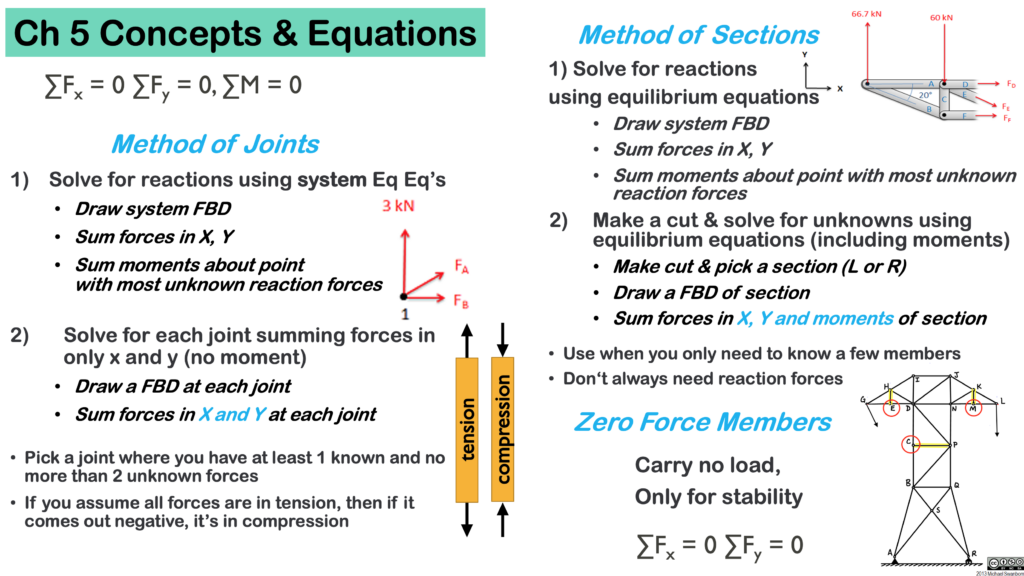# Chapter 5: Trusses

This chapter will introduce you to a special type of structure called a ‘truss’. You’ll analyze these structures more in your Structures course, but for Statics you will need to know how to calculate the force in each member, using two methods: method of joints and method of sections. At first this might seem confusing, but there is something quite elegant and magical about the method once you understand it. Here are the sections in this Chapter:

Here are the important equations for this chapter: